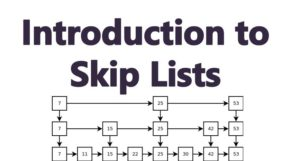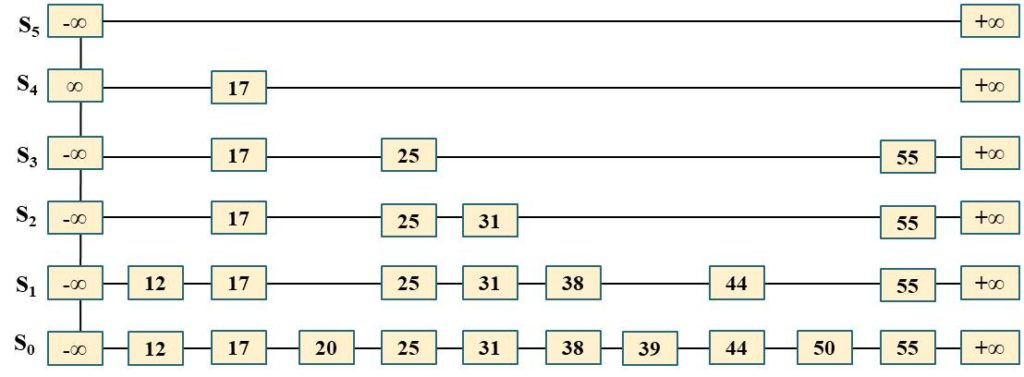# Skip Lists and How They Work

A skip list is an efficient data structure  that arranges the elements in a list in such a way that the search and update takes O(nlogn) time on average. n is the number of items in the list.

We would cover the following##### 1. How Skip Lists Work

First of all, we define a skip list S for a map M consists of a series of lists {S0, S1,  S2, . . . , Sh)

Each list Si in the set maintains a subset of the elements of M which is sorted in increasing order of the keys. Additionally, each list Si also stores two special keys, denoted as -∞ and +∞ where -∞ is smaller than all possible keys that can be inserted in m and +∞ is greater than all possible keys that can be inserted in M. Additionally, the sublists satisfy the following conditions:

• List S0 contains every possible item that can be stored in the map M (plus  -∞ and +∞).
• For i = 1, . . . h-1, Si contains a randomly generated subset of the entries of Si-1
• Lists Sh contains only  -∞ and +∞,

##### 2. Skip List Example

An example of a skip list is given in Figure 1.0. In this skip lists 10 items are stored in addition to -∞ and +∞.  S0 is at the bottom of the lists with all the possible elements  and as we move to the top of the list from S0 to Sh, we could see that the number of items gradually reduces such that Si+ 1 < Si and also Si+1 ⊂ Si (Si+1 is a subset of Si)Figure 1.0: An example of a skip lists that stores 10 entries and maintains 6 sublists: S0 to S5 and a height of 6

The insertion into a skip list is such that entries into sublist Si+1 is randomly chosen from the entries of Si with a probability of 1/2.  This means that the number entries of S1 is expected to be n/2. Similarly, the number of entries of S2 is expected to be n/4 and so on.

Generally, you can expect the number of entries in Si to be 1/2i.  This would then mean that the expected height of the skip list would be log n.

We can therefore visualize skip lists as a 2-dimensional set of positions arranged horizontally in levels and vertically in towers. Each level is a sublist Si and each tower is a position for storing similar entries across the lists from bottom to top as can be seen in Figure 1.0. Maybe you can take some time to understand how it works

##### 3. Skip Lists Traversal

We can  all the positions in skip list by using the following set of operations:

• next(p): returns the position following p on the same level
• prev(p): returns the position preceding  p on the same level
• below(p): returns the position directly below p in the same tower
• above(p): returns the position directly above p in the same tower

If however any of the operations mentioned above are carried out and the position does not exist, then the operation returns a null as a result

##### 4. Skip List Implementation

You can implement a skip list using a linked structure that allows the four operations outlined above to each take constant time, O(1) given a skip list position p. Such linked list is a collection of doubly-linked list which of length h and  are aligned at the towers. The towers are themselves also doubly-linked list.

It is noteworthy that , in Java programming language, there is an implementation of the skip list data structure which is  available in the ConcurrentSkipListMap class which provides method for get(), put() and remove, operations which are guaranteed to be performed at O(log n) times.

Finally, The main advantage of the skip list is randomization, which makes them very simple and efficient.

In the next lesson, we would then  examine lookup and insertion into linked list.

##### Check these Data Structure and Algorithms Tutorials

Skip Lists and How They Work

Perfect Hashing – How it Works

Linear Probing, Quadratic Probing and Double Hashing

Universal Hashing

Search Time Under Simple Uniform Hashing

Hash Tables – Hashing With Chaining

Introduction to Hash Tables and Direct Addressing

Recurrences in Divide and Conquer Algorithm

How Bloom Filters Work

How Cuckoo Hashing Works

Introduction to AVL Trees (With Examples)#### kindsonthegenius

Kindson Munonye is currently completing his doctoral program in Software Engineering in Budapest University of Technology and Economics

View all posts by kindsonthegenius →